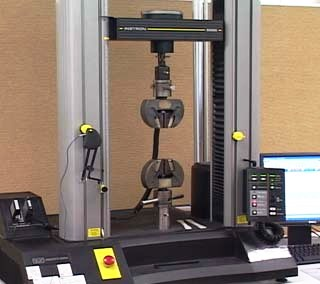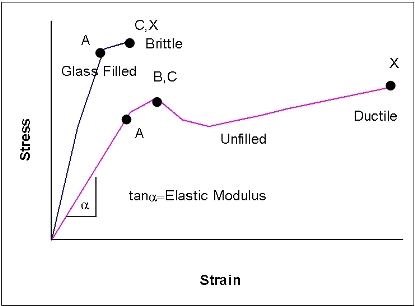# TENSILE STRENGTH, STRAIN AND MODULUSThe purpose of this test is to give an indication of a material’s performance under load. The given load is defined as the stress and the amount of deformation is known as the strain. By knowing the amount of strain a designer can have some idea of how a particular product or component will perform under working conditions.

Tensile testing involves stretching a specimen and measuring the load carried. The load and deflection data are translated into a stress-strain curve from which a range of tensile properties can be extracted. This is short term data obtained over a limited time period and usually under ideal laboratory conditions. Due to the majority of plastic materials being sensitive to temperature, the likely working conditions for the product or component need to be borne in mind when studying performance data.### TEST PIECES

Test specimens vary in size from 165mm to 246mm for the length and 6mm to 19mm for the width of the narrow part. There is also a smaller version of 63.5mm length and 3.2mm width (ASTM D638 Type IV and ISO527 5A and 5B). Thicknesses also vary.

The size of bar used depends on different requirements. For the ASTM tests there is a preferred specimen size, if this does not break under testing then an alternative size is selected. Other sizes are used for comparisons of materials with different levels of rigidity. The small specimen is used when available material or laboratory space is limited.

For the ISO tests there is a preferred size for moulded specimens and a different size for machined. There are also different speeds for testing tensile modulus and also for testing of strain for unfilled and glass-filled polymers. The variations in sample sizes clearly show why it is not possible to accurately assess the differences between materials but they do provide a basis for initial screening and selection of materials.### ASTM D638

Property Definition
Tensile Elongation at Break Tensile elongation corresponding to the point of rupture
Tensile Elongation at Yield Tensile elongation corresponding to the yield (an increase in strain does not result in an increase in stress)
Tensile Strength at Break Tensile stress corresponding to the point of rupture
Tensile Strength at Yield Tensile stress corresponding to the yield point (an increase in strain does not result in an increase in stress)
Tensile Strength Tensile stress at a specified elongation
Tensile Strength, Ultimate The highest tensile stress a material can support before failing
Tensile Modulus The ratio of tensile stress to tensile strain of a material in the elastic region of a stress-strain curve.  A “Tangent” tensile modulus value is the slope of the elastic region of the stress-strain curve and is also known as Young’s Modulus, or the Modulus of Elasticity.  A “Secant” tensile modulus value is the slope of a line connecting the point of zero strain to a point on the stress-strain curve at a specified strain.  This is used for materials that exhibit little or no linear behaviour.

### ISO 527

Property Definition
Tensile Strain at Break Tensile strain corresponding to the point of rupture
Nominal Tensile Strain at Break Tensile strain at the tensile stress at break
Tensile Strain at Yield Tensile strain corresponding to the yield (an increase in strain does not result in an increase in stress)
Tensile Stress at Break Tensile stress corresponding to the point of rupture
Tensile Stress at 50% Strain Tensile stress recorded at 50% strain
Tensile Stress at Yield Tensile stress corresponding to the yield point (an Increase in strain does not result in an increase in stress)
Tensile Modulus Often referred to as Young’s Modulus, or the modulus of elasticity, tensile modulus is the slope of a secant line between 0.05% and 0.25% strain on a stress-strain plot.### STRESS-STRAIN CURVES EXPLAINED

Letter Term Definition
A Proportional Limit Maximum load (stress) the material is capable of sustaining without deviation from the proportionality (linearity) of stress to strain
B Yield Point The first stress value on a curve where an increase in strain occurs without an increase in stress.  Not all plastic materials exhibit a yield point
C Ultimate Strength The maximum stress a material will withstand when subjected to an applied strain.
X Break The strain value associated with breaking strength.
Elastic Limit The maximum stress a material can withstand and still recover (with no permanent deformation) to its original dimensions when the stress is released.
0 to A Yield region, elastic behaviour
Beyond A Plastic behaviourGET A QUOTE